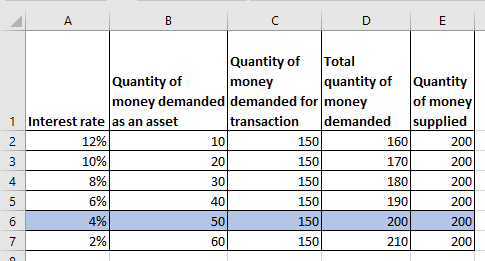# Quiz 33: Money Creation

Business

Given that, the money supply is \$200 billion, quantity of money demanded for transactions is \$150 billion, quantity of money demanded as an asset is \$10 billion at 12% interest increasing by \$10 billion for each 2% fall in the interest rate. (a) Below table shows the calculation of the interest rate:Here, total quantity of money demanded is sum of the quantity of money demanded as an asset and transaction. The equilibrium interest rate in country T is at the point where the quantity of money demanded is equal to the quantity of money supplied. Therefore, from the above table it can be observed that the equilibrium interest rate at country T is 4%. (b) At the equilibrium interest rate of 4% the total quantity of money demanded as well as the quantity of money supplied are \$200 billion. The amount of money demanded for transactions is \$150 billion , and the amount of money demanded as an asset is \$50 billion.

There is no answer for this question

There is no answer for this question

There is no answer for this question

There is no answer for this question

There is no answer for this question